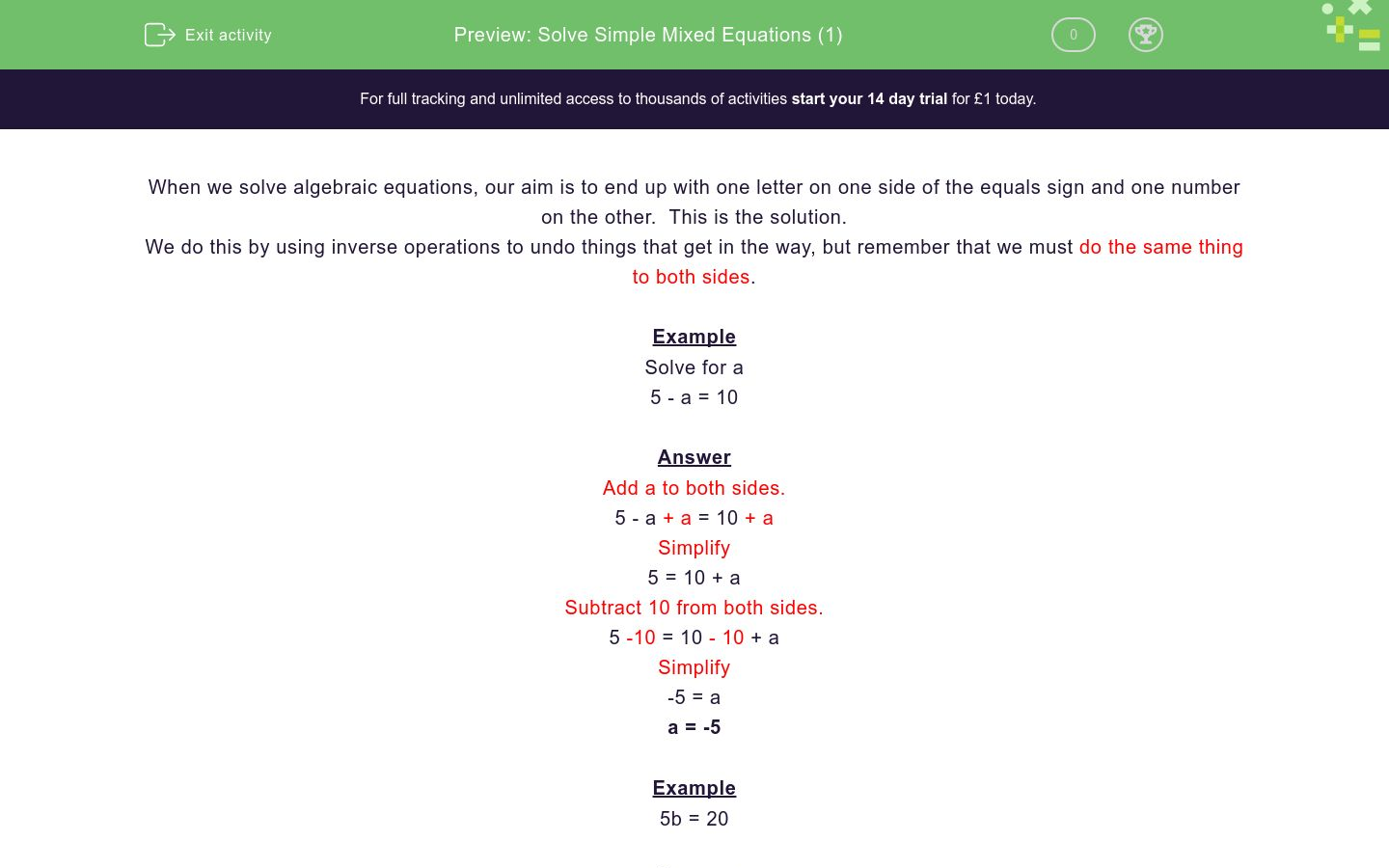# Solve Simple Mixed Equations (1)

In this worksheet, children must solve simple one-stage equations.Key stage:  KS 3

Curriculum topic:   Algebra

Curriculum subtopic:   Solve Linear Equations (One Variable)

Difficulty level:### QUESTION 1 of 10

When we solve algebraic equations, our aim is to end up with one letter on one side of the equals sign and one number on the other.  This is the solution.

We do this by using inverse operations to undo things that get in the way, but remember that we must do the same thing to both sides.

Example

Solve for a

5 - a = 10

5 - a + a = 10 + a

Simplify

5 = 10 + a

Subtract 10 from both sides.

5 -10 = 10 - 10 + a

Simplify

-5 = a

a = -5

Example

5b = 20

Divide both sides by 5

5b ÷ 5 = 20 ÷ 5

Simplify

b = 4

Example

b ÷ 5 = 20

Multiply both sides by 5

b ÷ 5 x 5 = 20 x 5

Simplify

b = 100

Solve for a:

7 + a = 15

a = 8

8

a = 22

Solve for a:

a - 13 = 15

a = 28

a = -2

a = 2

Solve for a:

7a = 21

a = 28

a = 3

a = 14

Solve for a:

a ÷ 3 = 21

a = 24

a = 7

a = 63

Solve for a:

a - 28 = 21

a = 49

a = -7

a = 7

Solve for a:

18 - a = 24

a = 6

a = 42

a = -6

Solve for a:

a ÷ 3 = 18

a = 54

a = 6

a = -3

Solve for a:

3a = 18

a = 54

a = 6

a = 15

Solve for a:

15 - a = 13

a = 12

a = -28

a = 2

Solve for a:

15a = -30

a = -2

a = -15

a = 15

• Question 1

Solve for a:

7 + a = 15

a = 8
• Question 2

Solve for a:

a - 13 = 15

a = 28
• Question 3

Solve for a:

7a = 21

a = 3
• Question 4

Solve for a:

a ÷ 3 = 21

a = 63
• Question 5

Solve for a:

a - 28 = 21

a = 49
• Question 6

Solve for a:

18 - a = 24

a = -6
• Question 7

Solve for a:

a ÷ 3 = 18

a = 54
• Question 8

Solve for a:

3a = 18

a = 6
• Question 9

Solve for a:

15 - a = 13

a = 2
• Question 10

Solve for a:

15a = -30